# Week 8 - lecture 2

## TOP TOPIC

Date: 01/09/22

8.3. Lower bound for the trace of a transition matrix
8.4. The 3-1 tree
8.5. Proving fast mixing for Potts model via contraction
8.6. Swendsen-Wang dynamics and the random cluster model

## Homework

1.* Letbe an irreducible transition matrix on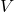, and suppose thatis nonzero and satisfies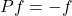.

(a) Show that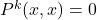for all odd.

(b) Deduce that there is a partition ofinto two sets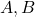such that if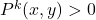, then either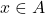and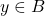, or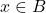and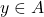.

2.* Letbe an irreducible transition matrix on, and suppose that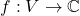is a nonzero complex eigenfunction,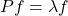, where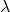may be complex and. Show that there is some positive integersuch that. Deduce that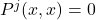for all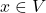, provided that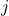is not divisible by.

2.* Consider the Potts model on an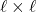square in the lattice. Show that if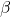is a large enough constant, then the mixing time of Glauber dynamics for this Potts model is at least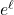.

3. Consider the Potts model on anvertex graph of maximal degree five. Show that if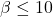,  then there is an absolute constantso that the mixing time of Glauber dynamics for this Potts model is at most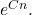## Reference

 Glasner, Shmuel.
Almost periodic sets and measures on the torus.
Israel J. Math. 32 (1979), no. 2-3, 161–-172.

 Berend, Daniel and Peres, Yuval.
Asymptotically dense dilations of sets on the circle.
J. London Math. Soc. (2) 47 (1993), no. 1, 1–-17.

 N. Alon and Y. Peres, "Uniform dilations'', Geometric and Functional Analysis, vol. 2, No. 1 (1992), 1–28.

 Nair, R. and Velani, S. L.
Glasner sets and polynomials in primes.
Proc. Amer. Math. Soc. 126 (1998), no. 10, 2835–-2840.